# LOG#038. Relativity: Examples(II).

Example 1. Completely inelastic collision of 2 particles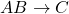.

We will calculate the mass and velocity after the collision, when a cluster is formed.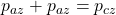From the consevation of momentum in the collision, we get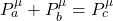Then,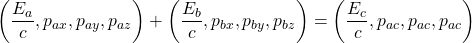Squaring both sides: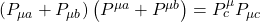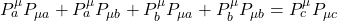In the rest frame of the particle labelled with “a”: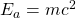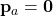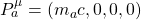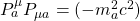Now, withand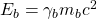and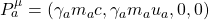And thus,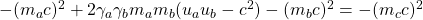From this equation, we get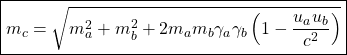andIf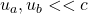, then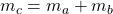, as we should expect from classical physics ( Lavoisier’s law: the mass is conserved).

We observe that, in general, there is NO rest mass conservation law in relativistic physics ( as we have mentioned before). Momentum and energy can be transformed into rest mass and viceversa. From the next equations: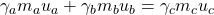We getis the center or mass velocity in the laboratory frame. If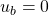and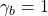, thenAnd if we write, the formula provides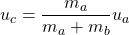And it is the classical center of mass equation for two particles, as we expected to be.

Example 2. Production of a proton-antiproton pair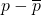in the chemical highly energetic process given by.

If we get an extremely energetic beam of protons. Then, we collide one proton A of the beam with another proton B, at rest. If the kinetic energy is enough, we can create a proton-antiproton pair! The question is, what is the minimum (total) energy, sometimes called threshold energy?What is the minimum kinetic energy, or threshold kinetic energy? And what is the velocity of the incident proton A in order to get the pair production? We are going to solve this really nice problem.

The proton B at rest has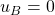and then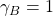.

Note that we have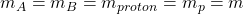and, for the system after the collision when the pair is created.

Using the formulae we have studied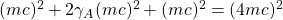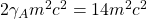and thus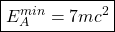It impliesfor the kinetic threshold energy. The necessary velocity can be obtained in a simple way:And thus,Energetically, we find that the collision is more “favourable”, in general, when we get a storage ring with 2 protons and we crash them with. In that case, the energy threshold and the kinetic energy threshold, provide: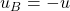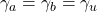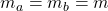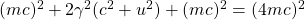and then, the minimum velocity of each proton has to be:Thus, the minimun threshold total energy of each proton is:Therefore, the minimum kinetic energy, the kinetic threshold energy, is given by. For each proton PAIR, the total energy threshold is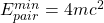. This value differs from the relativistic value previously obtained by an amount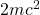. Now the kinetic threshold energy to obtain the pair equals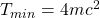i.e., 1/3 ()of the total energy in the previous example () is saved smashing 2 protons in opposite directions in order to create the pair proton-antiproton.

We can generalize the problem to the creation of n-pairs proton-antiproton. The equations yield:and then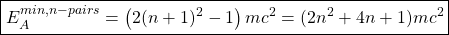The threshold kinetic energy will be in this case:and the incident minimum velocity to hit the proton at rest is now:In the case of a very large number of pairs,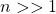we get that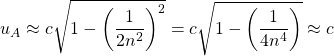In the case of a storage ring,and from this equation, algebra providesandThis site uses Akismet to reduce spam. Learn how your comment data is processed.